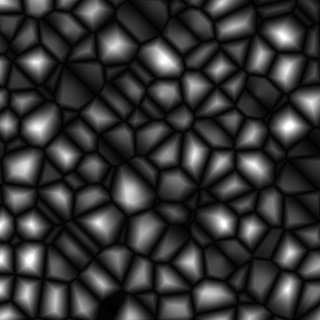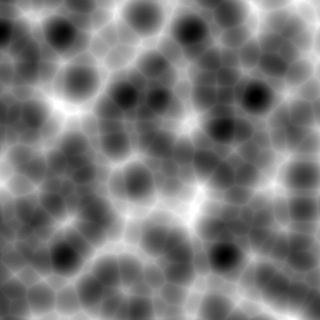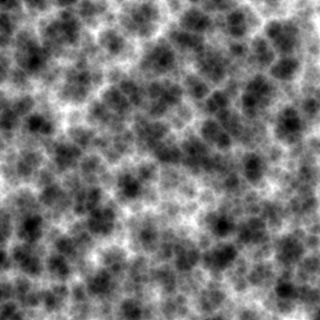## 2007.10.27 - Jpeg Buoys Amidst a Sea of Text

So I put off working on this entry long enough that it’s now two entries worth of data in one.

#### Too Many Instructions: Cutting Down On the Noise

So, the implementation of Improved Perlin noise from GPU Gems 2 boils down to 48 pixel shader instruction slots (9 texture, 39 arithmetic). That’s one octave of noise. What I needed, desperately, was a faster implementation of noise, where the base quality doesn’t matter (especially useful for things such as fBm and the like).

In the FIRST GPU Gems, in the chapter on Improved Perlin Noise, Ken Perlin makes a quick note about how to make a cheap approximation of perlin noise in the shader, using a volume texture. The technique is straight forward, but it took me some effort to understand exactly what was supposed to go into the volume texture.

In my case, I ended up using a 32x32x32 volume texture to simulate an 8x8x8 looping sample of perlin noise space. Essentially, when sampling this texture, divide the world position by 8, and use that as the (wrapped) texcoord into the volume.

#### Crazy 8s: Modifying Perlin Noise To Loop At A Specified Location

The first trick is that it has to be LOOPING Perlin noise. But how do you generate such a thing?

Turns out, in the reference implementation of Improved Noise, there are a bunch of instances where there are +1s. For instance:

```A = p[X  ]+Y;
AA = p[A]+Z;
AB = p[A+1]+Z;

B = p[X+1]+Y;
BA = p[B]+Z;
BB = p[B+1]+Z;```

(Later, AA, AB, BA, and BB are also accessed with +1s).

Figuring out how to make the noise wrap at a specific value (in my case, 8), was a matter of rethinking those as follows:

```A = p[X  ]; // note: no +Y here
AA = p[A+Y]  (+Z); // +Z in parens because it actually gets added later, like the Y does here
AB = p[A+(Y+1)] (+Z);

B = p[X+1]; // again, no +Y
BA = p[B+Y] (+Z);
BB = p[B+(Y+1)] (+Z);```

So, really, the +1s are added to the coordinate added earlier.
So, to make the noise wrap at a certain value, you need to take those (coordinate+1)s and change each into a ((coordinate+1)%repeatLocation).

The final version of the texture shader that generates noise that loops at a specific location is as follows:

```// permutation table
static int permutation[] = { 151,160,137,91,90,15,
131,13,201,95,96,53,194,233,7,225,140,36,103,30,69,142,8,99,37,240,21,10,23,
190, 6,148,247,120,234,75,0,26,197,62,94,252,219,203,117,35,11,32,57,177,33,
88,237,149,56,87,174,20,125,136,171,168, 68,175,74,165,71,134,139,48,27,166,
77,146,158,231,83,111,229,122,60,211,133,230,220,105,92,41,55,46,245,40,244,
102,143,54, 65,25,63,161, 1,216,80,73,209,76,132,187,208, 89,18,169,200,196,
135,130,116,188,159,86,164,100,109,198,173,186, 3,64,52,217,226,250,124,123,
5,202,38,147,118,126,255,82,85,212,207,206,59,227,47,16,58,17,182,189,28,42,
223,183,170,213,119,248,152, 2,44,154,163, 70,221,153,101,155,167, 43,172,9,
129,22,39,253, 19,98,108,110,79,113,224,232,178,185, 112,104,218,246,97,228,
251,34,242,193,238,210,144,12,191,179,162,241, 81,51,145,235,249,14,239,107,
49,192,214, 31,181,199,106,157,184, 84,204,176,115,121,50,45,127, 4,150,254,
138,236,205,93,222,114,67,29,24,72,243,141,128,195,78,66,215,61,156,180
};

// gradients for 3d noise
static float3 g[] = {
1,1,0,
-1,1,0,
1,-1,0,
-1,-1,0,
1,0,1,
-1,0,1,
1,0,-1,
-1,0,-1,
0,1,1,
0,-1,1,
0,1,-1,
0,-1,-1,
1,1,0,
0,-1,1,
-1,1,0,
0,-1,-1,
};

int perm(int i)
{
return permutation[i % 256];
}

float3 texfade(float3 t)
{
return t * t * t * (t * (t * 6 - 15) + 10); // new curve
//	return t * t * (3 - 2 * t); // old curve
}

float texgrad(int hash, float3 p)
{
return dot(g[hash%16], p);
}

float texgradperm(int x, float3 p)
{
return texgrad(perm(x), p);
}

float texShaderNoise(float3 p, int repeat, int base = 0)
{
int3 I = fmod(floor(p), repeat);
int3 J = (I+1) % repeat.xxx;
I += base;
J += base;

p -= floor(p);

float3 f = texfade(p);

int A  = perm(I.x);
int AA = perm(A+I.y);
int AB = perm(A+J.y);

int B  =  perm(J.x);
int BA = perm(B+I.y);
int BB = perm(B+J.y);

return lerp( lerp( lerp( texgradperm(AA+I.z, p + float3( 0,  0,  0) ),
texgradperm(BA+I.z, p + float3(-1,  0,  0) ), f.x),
lerp( texgradperm(AB+I.z, p + float3( 0, -1,  0) ),
texgradperm(BB+I.z, p + float3(-1, -1,  0) ), f.x), f.y),
lerp( lerp( texgradperm(AA+J.z, p + float3( 0,  0, -1) ),
texgradperm(BA+J.z, p + float3(-1,  0, -1) ), f.x),
lerp( texgradperm(AB+J.z, p + float3( 0, -1, -1) ),
texgradperm(BB+J.z, p + float3(-1, -1, -1) ), f.x), f.y), f.z);

}```

Whee!

#### Noise + Real Numbers + Imaginary Numbers == ???

So, the second trick: the texture actually needed to contain two values (R and G channels), to act as real and imaginary parts. Very simple, I added a base parameter (in the code above) so that I could offset into a different 8x8x8 cube of noise. I drop a different 8x8x8 noise into the G channel.

Finally! We have a texture with 8x8x8 noise. But 8-cubed noise sucks, because it’s ridiculously repetative. That’s where that weird imaginary part comes into play. You sample the 8-cube volume again, but at 9x scale (so it’s lower frequency). You then use the (real component of) high-frequency as an angle (scaled by 2pi) to do a quaternion rotation on the low-frequency noise.

```float noiseFast(float3 p)
{
p /= 8; // because the volume texture is 8x8x8 noise, divide the position by 8 to keep this noise in parity with the true Perlin noise generator.
float2 hi = tex3D(noise3dSampler, p).rg*2-1; // High frequency noise
half   lo = tex3D(noise3dSampler, p/9).r*2-1; // Low frequency noise

half  angle = lo*2.0*PI;
float result = hi.r * cos(angle) + hi.g * sin(angle); // Use the low frequency as a quaternion rotation of the high-frequency's real and imaginary parts.
return result; // done!
}```

And that’s it! Compare the instruction counts of the real Perlin noise to this fast fake:

```Old (high-quality):  approximately 48 instruction slots used (9 texture, 39 arithmetic)
New (lower-quality): approximately 20 instruction slots used (2 texture, 18 arithmetic)```

Essentially, wherever I don’t need the full quality noise, I can halve my instruction count on noise generation. Score!

Here’s a comparison: on the left, the weird confetticrete chair with the original noise, and on the right is the new faster noise:

They look roughly the same, there are some artifacts on the new one (the diamond-shaped red blob on the upper-right of the new chair due to the trilinear filtering), but it’s way faster.

#### Cellular Noise

Okay, I have some cool perlin noise stuff. But man cannot live on Perlin noise alone, so I decided to implement cellular noise, as well.

Turns out, there’s something called Worley noise which does exactly what I was hoping to do. Implementation was pretty simple.

```void voronoi(float3 position, out float f1, out float3 pos1, out float f2, out float3 pos2, float jitter=.9, bool manhattanDistance = false )
{
float3 thiscell = floor(position)+.5;
f1 = f2 = 1000;
float i, j, k;

float3 c;
for(i = -1; i <= 1; i += 1)
{
for(j = -1; j <= 1; j += 1)
{
for(k = -1; k <= 1; k += 1)
{
float3 testcell = thiscell  + float3(i,j,k);
float3 randomUVW = testcell * float3(0.037, 0.119, .093);
float3 cellnoise = perm(perm2d(randomUVW.xy)+randomUVW.z);
float3 pos = testcell + jitter*(cellnoise-.5);
float3 offset = pos - position;
float dist;
if(manhattanDistance)
dist = abs(offset.x)+abs(offset.y) + abs(offset.z);
else
dist = dot(offset, offset);
if(dist < f1)
{
f2 = f1;
pos2 = pos1;
f1 = dist;
pos1 = pos;
}
else if(dist < f2)
{
f2 = dist;
pos2 = pos;
}
}
}
}
if(!manhattanDistance)
{
f1 = sqrt(f1);
f2 = sqrt(f2);
}
}```

The gist is that each unit cube cell has a randomly-placed point in it. for each point being evaluated by the shader, you find the distance to the nearest point (a value called “F1”), and the distance to the next-nearest (“F2”), etc (to as many as you care about – though anything past F4 starts to look similar and uninteresting). Using linear combinations of these distances gives interesting results:Left: F2-F1 Right: (F1+F2)/2
Click to enlarge

Something cool to do, also, is to use Manhattan distance instead of standard Euclidian distance to calculate the distance. You end up with much more angular results. Here are the same 4 calculations, using manhattan distance:

Considering that a few levels of my current project will take place in a metallic fortress, this will especially come in handy.

So, what can you do with these?

I, predictably, have made a few test textures:

Also, it still looks pretty cool if you use fBm on it. For instance:4 octaves of F1 Worley noise

But I hear you asking “duz it wrok n 3deez, Drilian?!?!?!” Oh, I assure you it does!

And now I hear you asking “Can u stop typing nau? I is tir0d of reedin.” (or alternately, “I is tir0d uv looking @ imagez sparsely scattered thru the text taht I dun feel liek reedin.”) To this, I say: Sure, but it worries me that you’re asking your questions in some form of lolcat.

That’s all I got.

### 2 Responses to “Jpeg Buoys Amidst a Sea of Text”

1.Paulo Brito Says:

I would like to know if you have passed this procedural textures ( I m interested in cellulerB ) to XNA 3.1? It is possible to do that? I was viewing your code, and I m trying to run it ( need to install directx SDK) can I work with DirectX SDK august 2009?

The reason I want to play with procedural textures is because I need for a project related to multitouch table.

Thanks

Paulo Brito

2.Drilian Says:

As a matter of fact, yes – all of the textures in the game I’m working on right now are procedural.

You can generate them on the CPU into an array and then use Texture.SetData to copy them into video memory, or you can generate them using the GPU, rendering them to a RenderTarget (which is what I do).